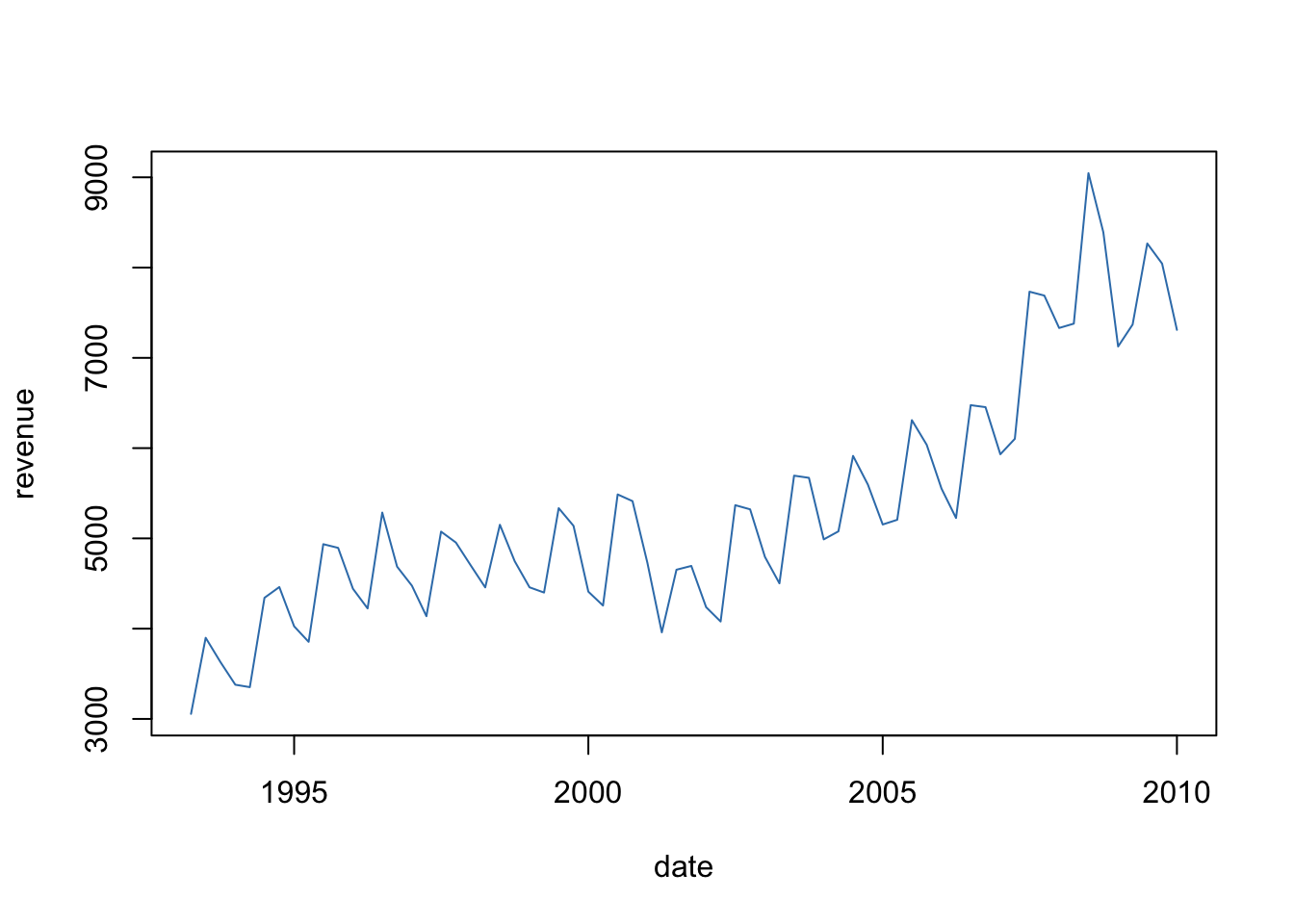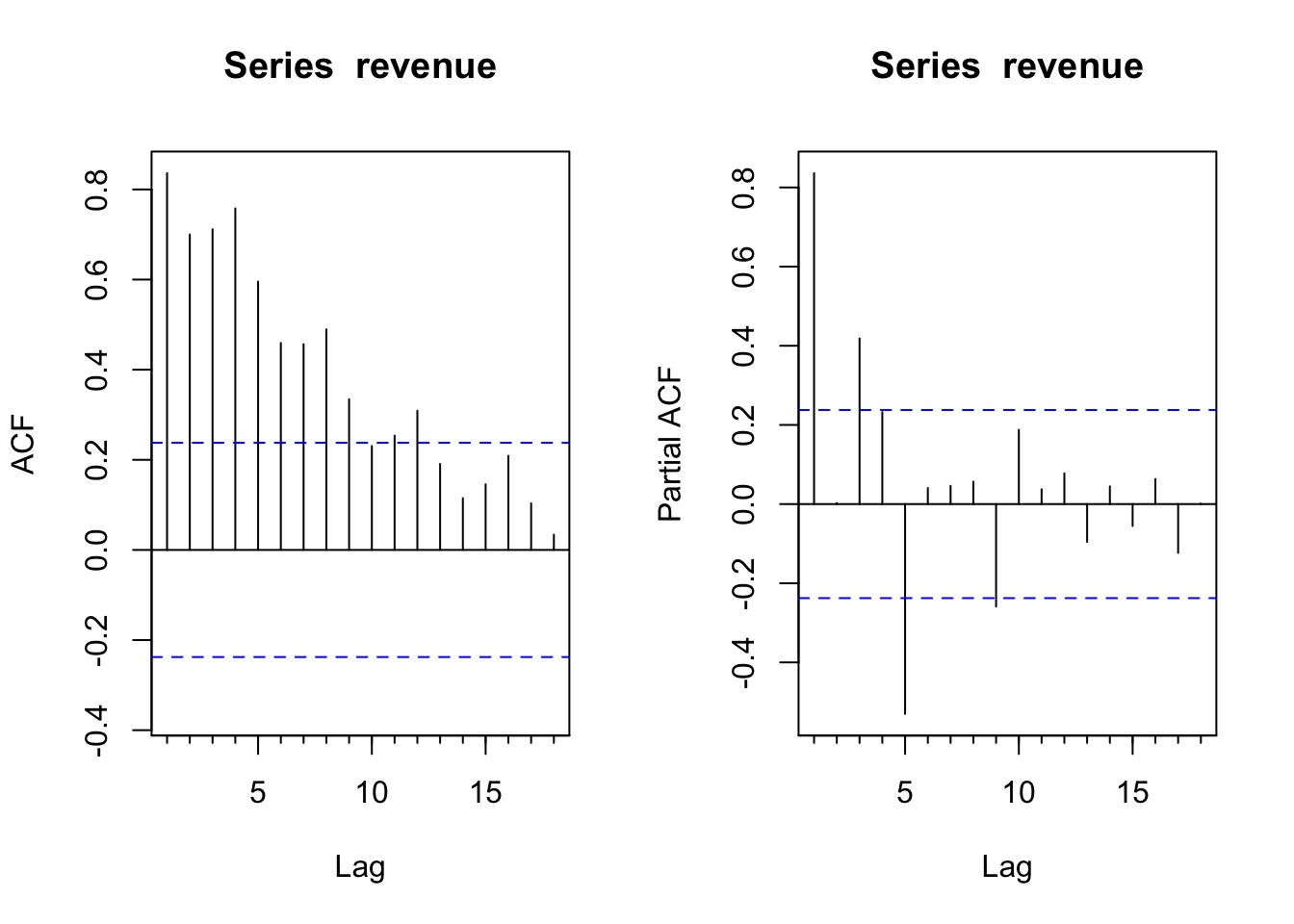## Preample

``library("forecast")``

## Coke Revenue

Coca-Cola Net Revenue, Quarterly, 1994-2009.

``````data <- read.csv("http://ptrckprry.com/course/forecasting/data/cokerev.csv")
date <- as.Date(data\$date)
time <- 1:length(date)
revenue <- data\$revenue``````

## Time Series Plots

``plot(date, revenue, type="l", col=2)``## ACF, PACF

Original Series

``````par(mfrow=c(1,2))
Acf(revenue)
Pacf(revenue)``````First Difference

``````par(mfrow=c(1,2))
Acf(diff(revenue))
Pacf(diff(revenue))``````